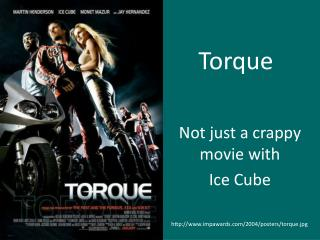DownloadDownload PresentationTorque

# Torque

Télécharger la présentation## Torque

- - - - - - - - - - - - - - - - - - - - - - - - - - - E N D - - - - - - - - - - - - - - - - - - - - - - - - - - -
##### Presentation Transcript

1. Torque Not just a crappy movie with Ice Cube http://www.impawards.com/2004/posters/torque.jpg

2. What Torque is • Torque is a force that produces a rotation • Think about using a wrench to turn a bolt • Torque depends on TWO THINGS: • How much force you apply • How far away from the center of rotation you apply it If FA and FB are equal, which will produce a greater torque in this example? http://upload.wikimedia.org/wikipedia/commons/2/21/Torque.JPG

3. Torque • The distance from the center of rotation to the location the force is applied is called the LEVER ARM. • In general, we care about the part of the force that is applied PERPENDICULAR to the lever arm Let’s say that the lever arm rotates around a pivot point to the left. If all three forces are the same magnitude, which one produces the greatest torque? F2 F1 Pivot F3 Lever arm

4. Torque: strange units • Torque is kind of like force, ‘cept different. • Force is a part of torque, but it also depends on that lever arm. • So torque is equal to force X length of the lever arm • Force is in Newtons, Length is in meters • So torque is given in Newton-Meters (or in foot-pounds if you are in the English system).

5. Perpendicular Force • It’s just like when we were talking about vectors • A force at an angle will have a component that is perpendicular to the lever arm. • What components of the forces below are perpendicular to the lever arms?

6. Torque brainstorm • Give some examples of torque that you use on a daily basis

7. Balanced Torques • Torques are forces (basically) and they can be in equilibrium with one another. • Example: kids on a teeter-totter (or seesaw or whatever the youth of today call these devices). • Weight provides force, seesaw is lever arm • Let’s say you and a friend are on either end of a seesaw. If you weigh the same amount, then how far should each of you sit from the pivot? • Now assume the unlikely scenario that the two of you are different weights. Where should you sit?

8. Torque example: IGNORE CRAZY UNITS http://microship.com/resources/resourcepix/cgdb-1.jpg

9. Rotational Inertia – Yes, it’s what you think • Remember inertia? Yeah, that was awesome. • Inertia was the tendency of an object in motion to stay in motion. • Likewise, rotational inertia is the tendency of an object that is rotating to keep rotating. • Just like it takes a force to change the linear state of motion, it takes a torque to change the rotational state of motion.

10. A subtle but important difference • For linear motion, inertia depends on mass • But rotational inertia depends not only on mass, but also on its DISTRIBUTION. • The closer in the mass is to the center of rotation… • The less rotational inertia • The easier it is to get rotating • The easier it is to stop rotating once it starts • The farther the mass is from the center of rotation… • The greater rotational inertia • The harder it is to get rotating • The harder it is to stop rotating once it starts

11. Examples and Demonstrations • “Choking up” on a baseball bat • Short Pendulum Vs. Long Pendulum • Animals (including you) running • Weights on a bar • Flipping pencil between fingers • Rolling hoops and disks • Gymnastics and other spinning people

12. Angular Momentum • Rotational inertia of an object X how fast it’s rotating • Angular momentum can be conserved • If you change the rotational inertia, the rotational speed will also change to compensate • Examples • Guy in chair with weights • Flywheels • Gymnasts